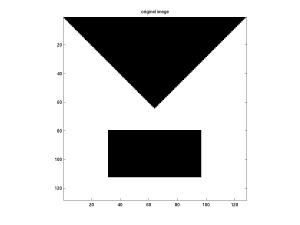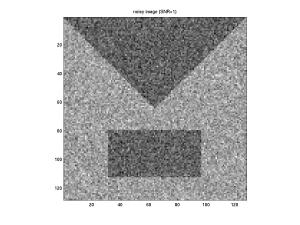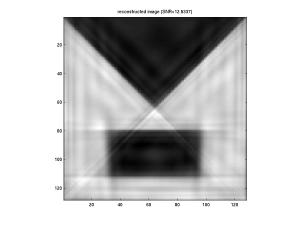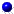Nonequispaced fast Fourier transform
Ridgelet transform
Ridgelet transform

# Ridgelet transform

The discrete ridgelet transform is designed by first using a discrete Radon transform based on the nonequispaced fast Fourier transform and then applying a dual-tree complex wavelet transform.
The Matlab script file ridgelet.m (see he folder ./examples/radon) contains an example of denoising by hard thresholding the discrete ridgelet coefficients of a noisy image. The discrete ridgelet transform is based on the fast discrete NFFT-based Radon and translationinvariant discrete Wavelet transform based on the free Matlab-Toolbox WaveLab850.

Left to right: orginal image, noisy image (SNR=1), and reconstructed image (SNR=12.55)The algorithms are implemented by Markus Fenn in `./applications/radon`. Related paper areFenn, M. and Ma, J.
Combined complex ridgelet shrinkage and total variation minimization.
SIAM J. Sci. Comput. 28, 984 - 1000 (2006)